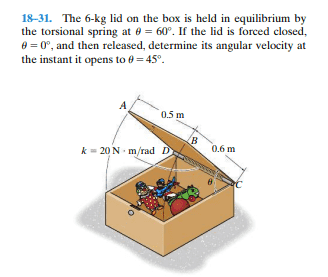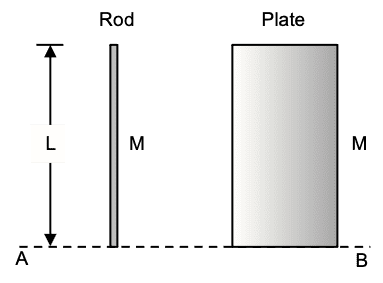# Rotating Rod in Plane: Kinetic Energy & Moment of Inertia

• simphys
In summary, the conversation discusses the concept of rotation around a fixed axis in a plane and how this can be applied to the kinetic energy and moment of inertia. The moment of inertia is a geometrical property that depends on the distribution of mass around the axis and is not affected by rotation or torque. The conversation also touches on the use of the principle of work and energy for rigid bodies and the parallel axis theorem for calculating the moment of inertia of a thin plate. Finally, the conversation discusses the use of Hooke's law for a torsion spring and how to find the spring constant.f

#### simphys

Homework Statement
The 6-kg lid on the box is held in equilibrium by
the torsional spring at theta = 60°. If the lid is forced closed,
theta = 0°, and then released, determine its angular velocity at
the instant it opens to theta = 45°.
Relevant Equations
ONLY PRINCIPLE OF WORK AND ENERGY USED!hello guys, I wanted to ask whether I can just consider/think about this as being rotation around a fixed axis in a plane representing it as if it was 'just' a rod. This is mainly so that for the kinetic energy in the second position is where if we think about it in just a plane. Is this correct? And then for the moment of inertia I'll take into account that it is a squared surface.

EDIT: can I get some help please on how to determine the angles that need to be used for the work done by the torsional spring, I don't know which to use in the equation of the work integral of between which angles?

Last edited:
Call the moment of inertia ##I## and put that in the energy conservation equation. What difference does it make if it is the moment of inertia of a rod or of a rectangular plate?

Mechanical energy conservation says that ME at point A is the same as ME at point B. Where do you think points A and B ought to be? Read the question.

Call the moment of inertia ##I## and put that in the energy conservation equation. What difference does it make if it is the moment of inertia of a rod or of a rectangular plate?

Mechanical energy conservation says that ME at point A is the same as ME at point B. Where do you think points A and B ought to be? Read the question.
Will the moment of intertia be the same then? and yeah but I can only use principle of work and energy here, haven't seen conserv of energy for rigid bodies yet.

Will the moment of intertia be the same then?
The same as what? The moment of inertia about a given axis is a geometrical property and does not depend on whether the object is rotating or whether a torque is acting on it about that axis. It only depends on how mass is distributed about that axis.

The same as what? The moment of inertia about a given axis is a geometrical property and does not depend on whether the object is rotating or whether a torque is acting on it about that axis. It only depends on how mass is distributed about that axis.
yes ? so what do you mean by that, I already said that I am taking into account the moment of ineratia of the whole plate right? because if I were to look at it in 2D it would just look as if it's a rod which it is not no?

You can consider the lid as a concentrated mass of 6 kg rotating at 0.3 m from the pivot.
The spring creates a moment about the pivot, the weight of the mass creates a different and variable one.

It seems that the moment of the spring is to be considered constant with respect to the opening angle, according to the wording of the problem, not sure.

http://hyperphysics.phy-astr.gsu.edu/hbase/mi.html#mig

yes ? so what do you mean by that, I already said that I am taking into account the moment of ineratia of the whole plate right? because if I were to look at it in 2D it would just look as if it's a rod which it is not no?

Use (or calculate) the moment of inertia of a thin plate and the parallel axis theorem.

yes ? so what do you mean by that, I already said that I am taking into account the moment of ineratia of the whole plate right? because if I were to look at it in 2D it would just look as if it's a rod which it is not no?
The figure below shows a rod of length ##L## and mass ##M##. Next to it is a plate of height ##L## and mass also ##M##. The two objects are very thin in the direction into the screen. The moment of inertia of the rod about the dotted line AB is ##I_{\text{rod}}=\frac{1}{3}ML^2.##

What is the moment of inertia of the plate about the same axis?•erobz
It seems that the moment of the spring is to be considered constant with respect to the opening angle, according to the wording of the problem, not sure.
For a torsion spring, Hooke's law is ##\tau=\kappa \theta## where ##\tau## is the torque, ##\kappa## is the (torsion) spring constant and ##\theta## is the angle. The elastic torque is proportional to the angle. You can find the spring constant from the equilibrium condition, as for any (linear) spring problem.

•Lnewqban
For a torsion spring, Hooke's law is ##\tau=\kappa \theta## where ##\tau## is the torque, ##\kappa## is the (torsion) spring constant and ##\theta## is the angle.
You mean ##\tau=-\kappa \theta## because it is a restoring torque.

You can find the spring constant from the equilibrium condition, as for any (linear) spring problem.

You mean τ=−κθ because it is a restoring torque.
The spring constant is provided in the problem.
What is not provided is the unstretched origin vis. $$\tau=-\kappa (\theta-\theta_0)$$

•haruspex
It tells you at what angle is the equilibrium position under the torque produced by the weight of the lid.

It tells you at what angle is the equilibrium position under the torque produced by the weight of the lid.
As @hutchphd notes, you cannot determine the constant from that without a second datum, such as the relaxed angle.

It seems that the moment of the spring is to be considered constant with respect to the opening angle
That doesn’t work. It could not be in equilibrium at an elevated angle.

Will the moment of intertia be the same then?
You can think of the plate as a lot of rods in parallel. Their moments of inertia, about the same axis, add up, just like their masses do.

...
That doesn’t work. It could not be in equilibrium at an elevated angle.
I stand corrected.

As @hutchphd notes, you cannot determine the constant from that without a second datum, such as the relaxed angle.
On the illustration it says $$\kappa= 20 Nm/rad$$ so you have what you need

On the illustration it says $$\kappa= 20 Nm/rad$$ so you have what you need
Yes, I was responding to the apparent implication in post #12 that it could be deduced from the equilibrium angle.

•hutchphd